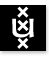Recursion Theory 2004/2005; 1st Semester Institute for Logic, Language & Computation Universiteit van Amsterdam

Instructor: Dr Benedikt L÷we, Dr Maricarmen MartĒnez
Vakcode: MolRT6
Time and place: Tuesday 13-15, P.014; Friday 11-13, P.018
Course language: English
Intended Audience: M.Sc. students of Logic and Mathematics
Prerequisites: This course assumes mathematical maturity and knowledge about first-order logic.

Content of the course:This lecture course will cover the basics of recursion theory (models of computation, limitative theorems) and discuss the connections between recursion theory and the foundations of mathematics (G÷del's Incompleteness Theorem). After that, recursion-theoretic hierarchies (Turing degrees) will be introduced.

Literature: Barry Cooper, "Computability Theory" (Chapters 1-10). We will sell the book to enrolled students for EUR 32 in the break of the first lecture on September 7, 2004 (at a substantial discount compared to the list price of \$69.95).

Organization: There will be 11 homework assignments for 6 points and one for 10 points, for a total of 76 points. The final grade will depend on the total number of homework points. A total of 40 points will be sufficient to pass the course. You can find your results from homework sets 1-7 listed by student ID here.

Topics and assignments:

• The topics covered and the assignments given in period a (week 1-8) are here.
• The topics covered and the assignments given in period b (week 9-16) are here.

Last update : November 9th, 2004